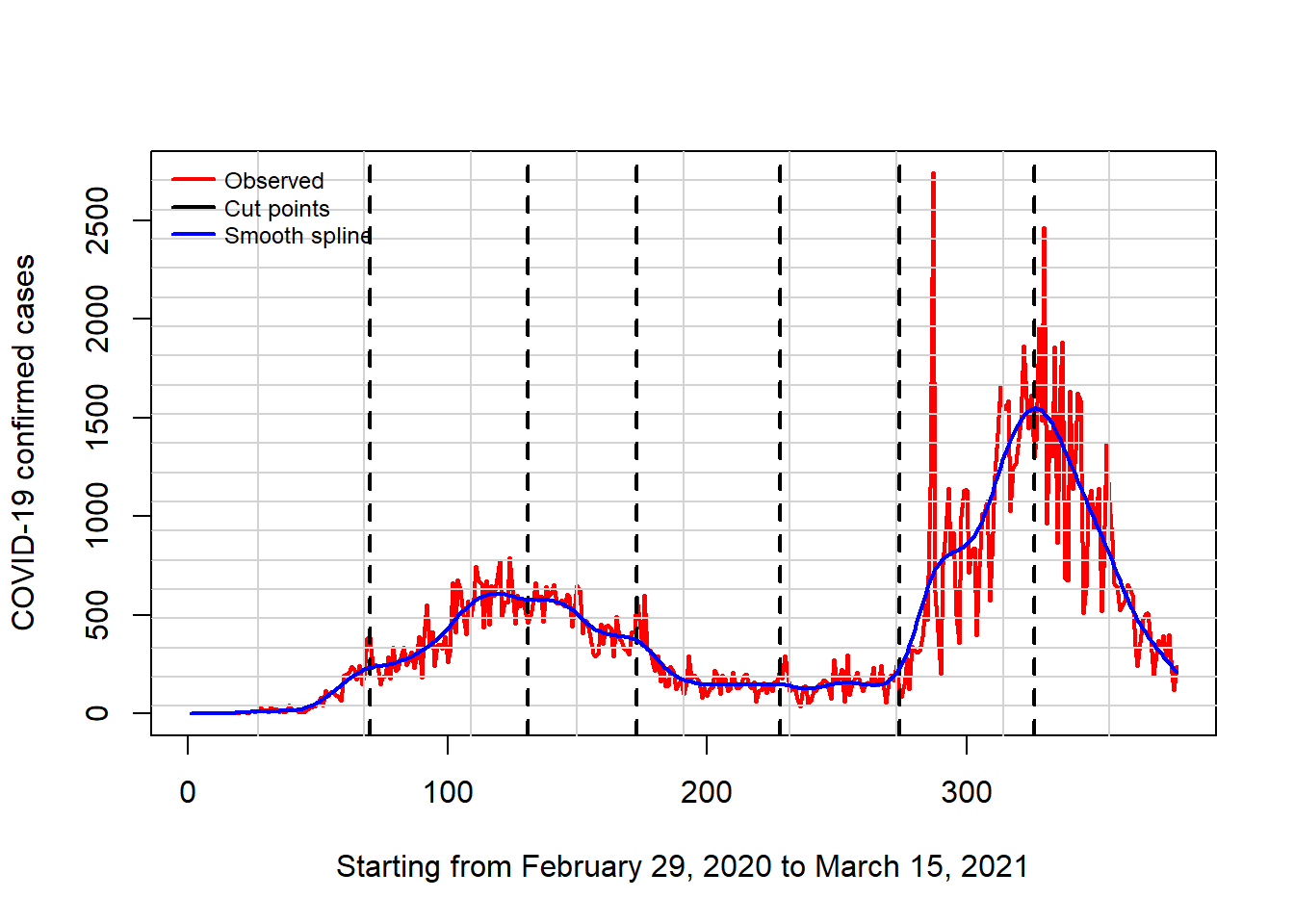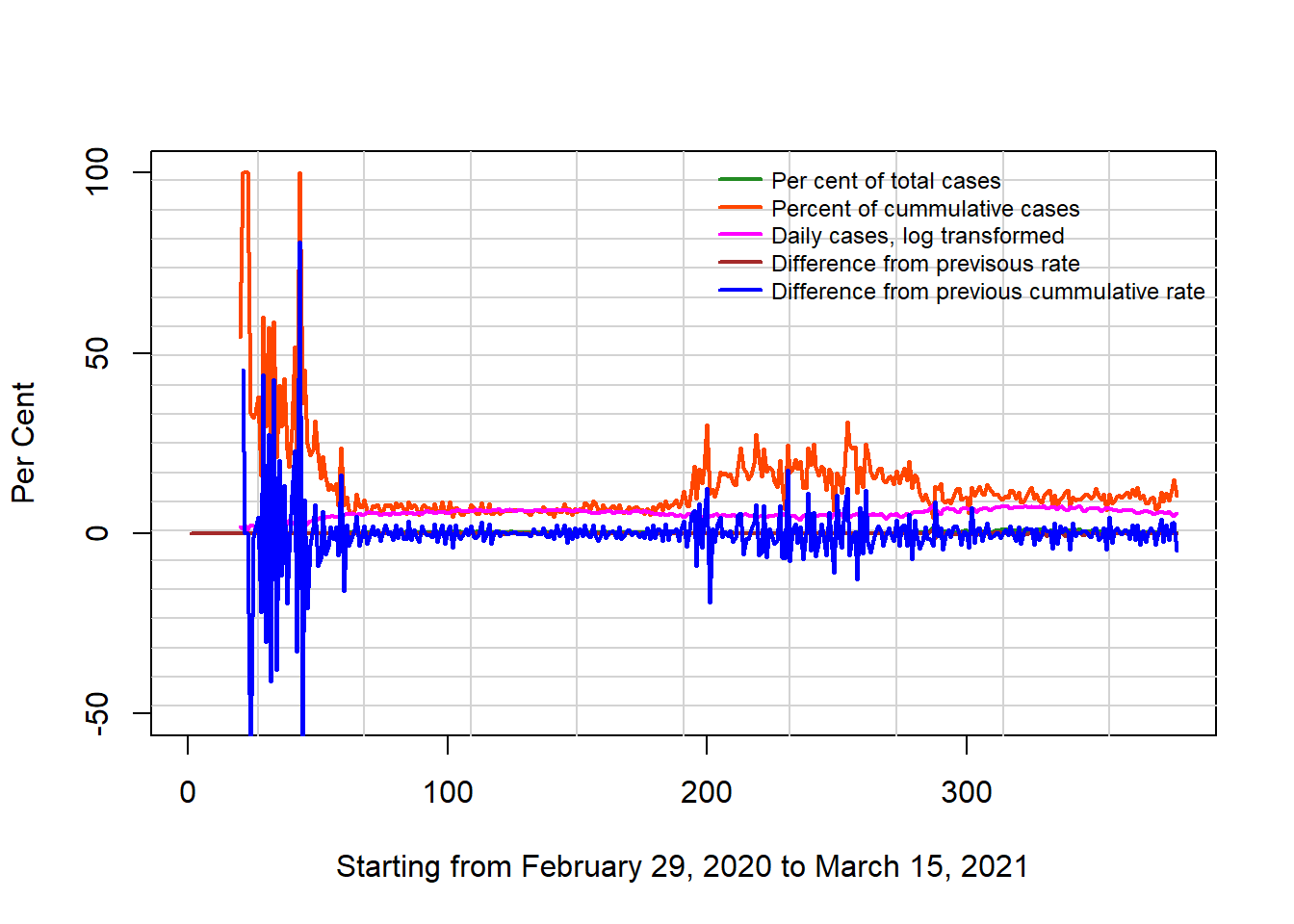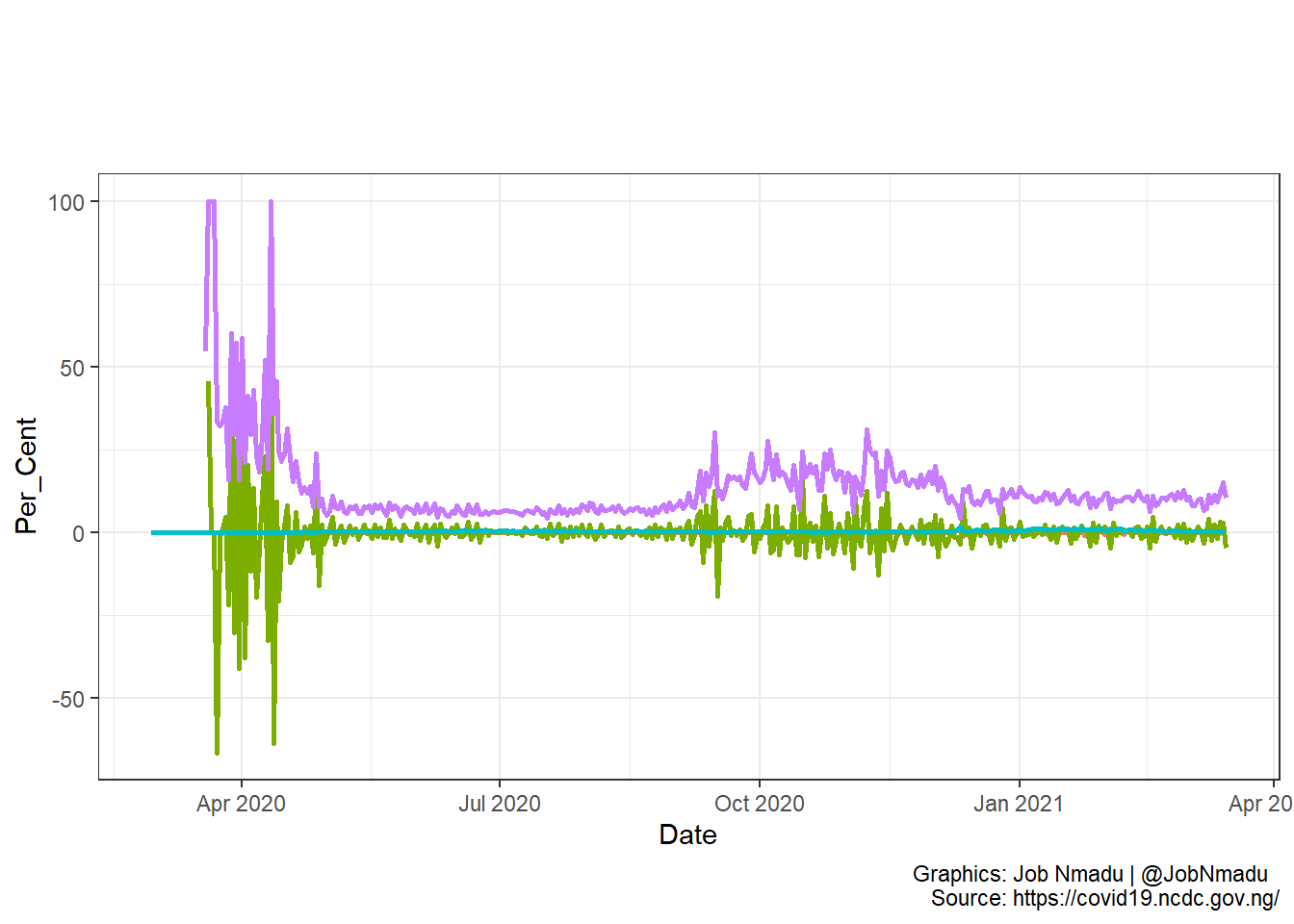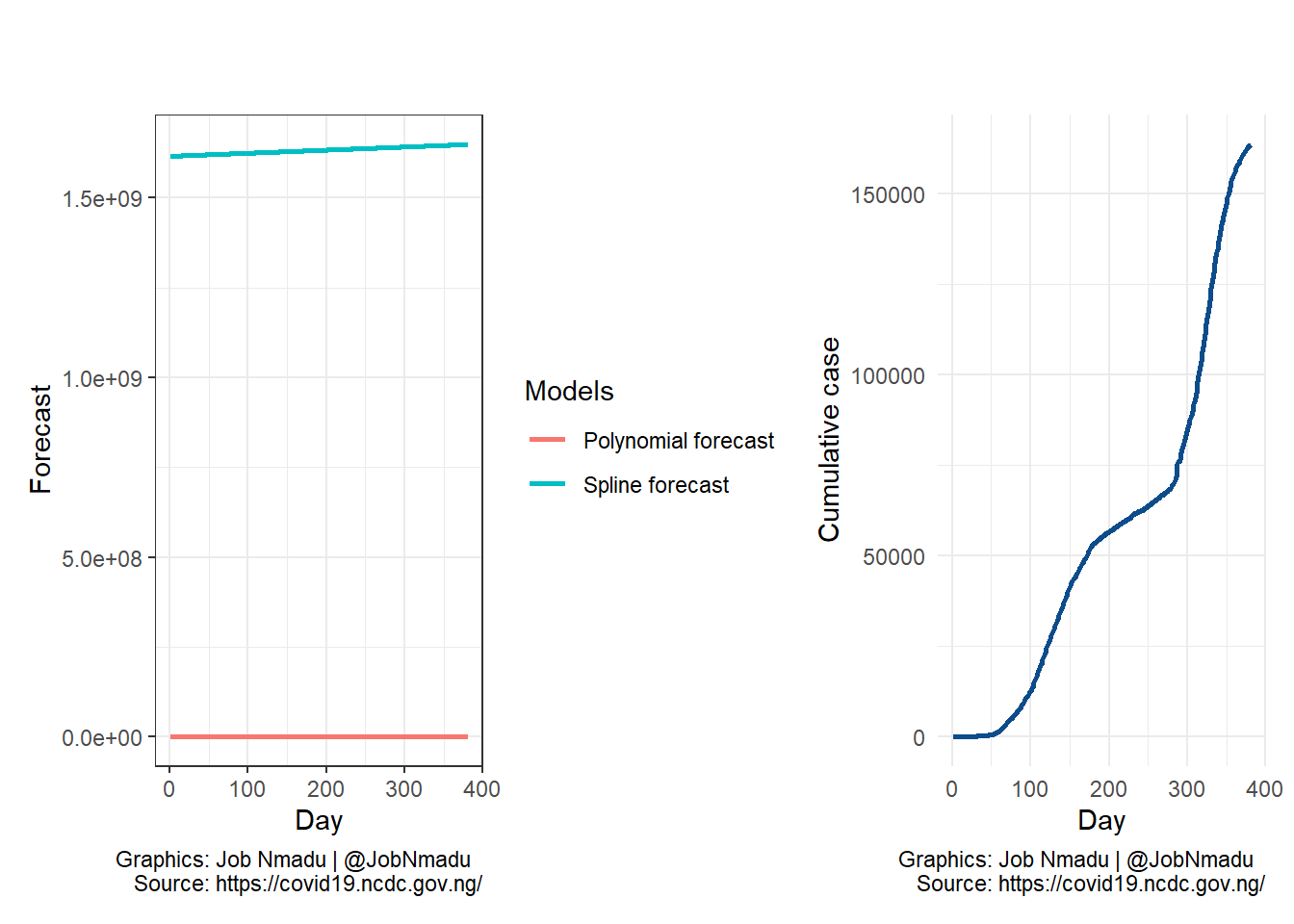# Load library and the data

The data is scrapped from the website of the Nigerian Centre for Disease Control (NCDC) i.e . The scrapping was done with some bits of tricks. Please see my post on that. The BREAKS were established from the visual inspection of the data (see )

library(tidyverse)
library(splines)
library(Metrics)
library(scales)
library(patchwork)
library(Dyn4cast)

BREAKS <- c(70, 131, 173, 228, 274, 326)

States_county <- read_excel("data/States.xlsx")

Like mentioned earlier, some tricks were used to obtain the daily data from the website of NCDC. The NCDC uploads the cumulative data on daily basis, so each day, the data were scrapped and then the daily incidence were recorded. For example, below is the data scrapped from the website on March 15, 2021.

Deaths <- readr::read_csv("data/today.csv")
names(Deaths) <- c("Region", "Confirmed", "Active", "Recovered", "Deaths")
DDmap <- Deaths
Deaths
## # A tibble: 37 × 5
##    Region      Confirmed Active Recovered Deaths
##    <chr>           <dbl>  <dbl>     <dbl>  <dbl>
##  1 Abia             1629     44      1564     21
##  2 Adamawa           942    641       270     31
##  3 Akwa Ibom        1707    483      1210     14
##  4 Anambra          1909     64      1826     19
##  5 Bauchi           1482    198      1267     17
##  6 Bayelsa           828     37       765     26
##  7 Benue            1188    575       591     22
##  8 Borno            1321     83      1200     38
##  9 Cross River       344     14       313     17
## 10 Delta            2593    780      1744     69
## # … with 27 more rows

# Creating date items

The dates are created starting with the day COVID-19 was first recorded in Nigeria, which is February 29, 2020. The sequence of dates were created fron the day to the last day the dataset was uploaded, which is March 15, 2021. In addition, sequence of dates were created from March 16, 2021 to march the number of days the virus have been recorded in Nigeria.

ss <- seq(1:length(cumper$DailyCase)) Dss <- seq(as.Date("2020-02-29"), by = "day", length.out = length(cumper$DailyCase))
Dsf <- seq(as.Date(Dss[length(Dss)] + lubridate::days(1)),
by = "day", length.out = length(cumper$DailyCase)) day01 <- format(Dss, format = "%B %d, %Y") daylast <- format(Dss[length(Dss)], format = "%B %d, %Y") casesstarts <- paste("Starting from", day01, "to", daylast, collapse=" ") lastdayfo <- format(Dsf[length(Dsf)], format = "%B %d, %Y") # Modelling and forecasting of COVID-19 caes The incidence of the virus was model using five machine learning algorithms which are: Splines model without Knots Smooth spline Splines model with Knots (or breaks) Quadratic Polynomial ARIMA Then using the ensemble technology, another set of four machine learning models were estimated as follows: Essembled based on summed weight Essembled based on weight of fit Essembled based on weight Essembled with equal weight The esemble technology is an attempt to combine models into one especially were the individual models are weak . fit0 <- lm(Case ~ bs(Day, knots = NULL), data = niz) fit <- lm(Case ~ bs(Day, knots = BREAKS), data = niz) fit1 <- smooth.spline(niz$Day, niz$Case) fita <- forecast::auto.arima(niz$Case)
fitpi <- lm(Case ~ Day + I(Day^2), data = niz)

kk  <- forecast::forecast(fitted.values(fit),
h = length(Dsf))
kk0 <- forecast::forecast(fitted.values(fit0),
h = length(Dsf))
kk1 <- forecast::forecast(fitted.values(fit1),
h = length(Dsf))
kk10 <- forecast::forecast(fitted.values(fitpi),
h = length(Dsf))
kk2 <- forecast::forecast(forecast::auto.arima(z.s$Case), h = length(Dsf)) kk30 <- (fitted.values(fit) + fitted.values(fit0) + fitted.values(fit1) + fitted.values(fitpi) + fita[["fitted"]])/5 kk31 <- forecast::forecast(kk30, h = length(Dsf)) kk40 <- lm(niz[,2]~fitted.values(fit)*fitted.values(fit0)* fitted.values(fit1)*fitted.values(fitpi)* fita[["fitted"]]) kk41 <- forecast::forecast(fitted.values(kk40), h = length(Dsf)) kk60 <- lm(niz[,2]~fitted.values(fit)+fitted.values(fit0)+ fitted.values(fit1)+fitted.values(fitpi)+ fita[["fitted"]]) kk61 <- forecast::forecast(fitted.values(kk60), h = length(Dsf)) KK <- as.data.frame(cbind("Date" = Dsf,"Day" = ss, "Without Knots" = kk[["mean"]], "Smooth spline" = kk0[["mean"]], "With Knots" = kk1[["mean"]], "Polynomial" = kk10[["mean"]], "Lower ARIMA" = kk2[["lower"]], "Upper ARIMA" = kk2[["upper"]])) KK <- KK[,-c(7,9)] names(KK) <- c("Date", "Day", "Without Knots", "Smooth spline", "With Knots", "Polynomial", "Lower ARIMA", "Upper ARIMA") WK <- sum(KK$Without Knots)
WKs  <- sum(KK$With Knots) SMsp <- sum(KK$Smooth spline)
LA   <- sum(KK$Lower ARIMA) UA <- sum(KK$Upper ARIMA)
POL  <- sum(KK$Polynomial) RMSE <- c("Without knots" = rmse(niz[,2], fitted.values(fit)), "Smooth Spline" = rmse(niz[,2], fitted.values(fit0)), "With knots" = rmse(niz[,2], fitted.values(fit1)), "Polynomial" = rmse(niz[,2], fitted.values(fitpi)), "Lower ARIMA" = rmse(niz[,2], fita[["fitted"]]), "Upper ARIMA" = rmse(niz[,2], fita[["fitted"]])) #RMSE <- 1/RMSE RMSE_weight <- as.list(RMSE / sum(RMSE)) KK$Date <- as.Date(KK$Date, origin = as.Date("1970-01-01")) DDf <- c("Without knots", "Smooth Spline", "With knots", "Quadratic Polynomial", "Lower ARIMA", "Upper ARIMA", "Essembled with equal weight", "Essembled based on weight", "Essembled based on summed weight", "Essembled based on weight of fit" ) KK$Essembled with equal weight <- kk31[["mean"]]
KK$Essembled based on weight <- kk41[["mean"]] KK$Essembled based on summed weight <- kk61[["mean"]]
ESS  <- sum(KK$Essembled with equal weight) ESM <- sum(KK$Essembled based on weight)
ESMS  <- sum(KK$Essembled based on summed weight) P_weight <- (fitted.values(fit) * RMSE_weight$Without knots) + (fitted.values(fit0) *
RMSE_weight$Smooth Spline) + (fitted.values(fit1) * RMSE_weight$With knots) + (fitted.values(fitpi) *
RMSE_weight$Polynomial) + (fita[["fitted"]] * RMSE_weight$Lower ARIMA)
kk51 <- forecast::forecast(P_weight, h = length(Dsf))
KK$Essembled based on weight of fit <- kk51[["mean"]] ESF <- sum(KK$Essembled based on weight of fit)
RMSE$Essembled with equal weight <- rmse(niz[,2], kk30) RMSE$Essembled based on weight <- rmse(niz[,2], fitted.values(kk40))
RMSE$Essembled based on summed weight <- rmse(niz[,2], fitted.values(kk60)) RMSE$Essembled based on weight of fit <- rmse(niz[,2], P_weight)
Forcasts <- colSums(KK[,-c(1,2)])

RMSE_f <- as.data.frame(cbind("Model" = DDf,
"Confirmed cases" =
comma(round(Forcasts, 0))))

Deaths$County <- States_county$County
Deaths <- Deaths[order(-Deaths$Deaths),] Deaths$Region <- factor(Deaths$Region, levels = Deaths$Region)
Deaths$Ratio <- Deaths$Deaths/Deaths$Confirmed*100 Deaths <- Deaths[order(-Deaths$Ratio),]
Deaths$Region <- factor(Deaths$Region, levels = Deaths$Region) Deaths$RecRatio <- Deaths$Recovered/Deaths$Confirmed*100
Deaths <- Deaths[order(-Deaths$RecRatio),] Deaths$Region <- factor(Deaths$Region, levels = Deaths$Region)
PerDeath <- round(sum((Deaths$Deaths)/ sum(Deaths$Confirmed)*100), 2)
PerRec <- round(sum((Deaths$Recovered)/ sum(Deaths$Confirmed)*100), 2)

RMSE_f$Recoveries <- comma(round(Forcasts * PerRec/100, 0)) RMSE_f$Fatalities <- round(Forcasts * PerDeath/100, 0)
RMSE_f$RMSE <- RMSE RMSE_f <- RMSE_f[order(-RMSE_f$Fatalities),]
RMSE_f$Model <- factor(RMSE_f$Model, levels = RMSE_f$Model) RMSE_f$Fatalities <- comma(RMSE_f$Fatalities) RMSE_fk <- knitr::kable(RMSE_f, row.names = FALSE, "html") # The report ### It is now 381 days since the first COVID-19 case was reported in Nigeria. As at March 15, 2021 the confirmed cases are 163,646 with 2,016 (1.25%, 100) fatalities, however, 145,752 (90.59%, 100) have recovered. ### Based on equal days forecast, by March 31, 2022, Nigeria’s aggregate confirmed COVID-19 cases are forecast to be: kableExtra::kable_styling(RMSE_fk, "striped") Model Confirmed cases Recoveries Fatalities RMSE Smooth Spline 889,385 805,694 11,117 371.3422 Upper ARIMA 805,328 729,547 10,067 209.3347 Quadratic Polynomial 598,274 541,977 7,478 373.6787 Essembled based on summed weight -70,387 -63,763 -880 185.4474 Essembled based on weight of fit -94,320 -85,444 -1,179 283.632 Essembled based on weight -182,959 -165,743 -2,287 179.7495 Essembled with equal weight -426,038 -385,948 -5,325 232.0987 Lower ARIMA -626,365 -567,424 -7,830 209.3347 Without knots -815,453 -738,719 -10,193 199.6519 With knots -1,114,856 -1,009,948 -13,936 187.3864 # Take a further look at some of the visuals plot(z.s[,1], z.s[,2], main = " ", col = "red", type = "l", lwd = 2, xlab = casesstarts, ylab = "COVID-19 confirmed cases") grid(10,20,lty=1,lwd=1) abline(v = BREAKS, lwd = 2, lty = 2, col = "black") #lines(density(z.s$Case), lwd = 2, type = "l", col = "blue", bw = 69.22)
lines(fitted.values(smooth.spline(z.s[,1],z.s[,2])), type = "l",
col = "blue", lwd = 2)
DDf <- c("Observed", "Cut points", "Smooth spline") #, "Density")
legend("topleft",
inset = c(0, 0),  bty = "n", x.intersp = 0.5,
xjust = 0, yjust = 0,
legend = DDf, col = c("red", "black", "blue"),
lwd = 2, cex = 0.75, xpd = TRUE, horiz = FALSE)The above plot shows that as at March 15, 2021; Nigeria has gone through two waves and there were more cases of COVID-19 in the second wave.

plot(cumper$Per, main = " ", xlab = casesstarts, ylab = "Per Cent", ylim = c(-50,100), type = "l", lwd = 2, col="forestgreen") grid(10,20,lty=1,lwd=1) lines(cumper$Per, lty=1,lwd=2, col= "forestgreen")
lines(cumper$PerCum, lty=1,lwd=2, col= "orangered") lines(log(cumper$DailyCase), lty=1,lwd=2, col= "magenta")
lines(cumper$LPer, lty=1,lwd=2, col= "brown") lines(cumper$LPerCum, lty=1,lwd=2, col= "blue")
legend("topright",
inset = c(0, 0, 0, 0),  bty = "n", x.intersp = 0.5,
xjust = 0, yjust = 0,
"topright", col = c("forestgreen", "orangered", "magenta", "brown", "blue"),
c("Per cent of total cases","Percent of cummulative cases",
"Daily cases, log transformed",
"Difference from previsous rate",
"Difference from previous cummulative rate"),
lwd = 2, cex = 0.75, xpd = TRUE, horiz = FALSE)The above two visuals show the log transformation of the distribution and the difference from previous day. The plots were mage with plot and ggplot functions respectively.The plot on the right is the cumulative cases of COVID-19 recorded in Nigeria while on the left is the forecast using spline and polynomial models. The forecast is based on equal duation of the lenght of the data.